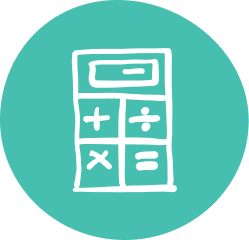### Quality Grade 10 Math Tutoring & Help

Oxford Learning® believes that learning is about more than just getting better grades. Our 10th Grade math tutoring programs help make sure your child has the skills to succeed in the classroom and beyond.

Oxford Learning®’s Grade 10 math tutoring program is specially designed to help students enhance their learning skills. This gives an excellent understanding of all the components of Grade 10 math while igniting a passion for learning. Our Grade 10 math tutors not only teach teens math concepts, but also teach teens how to think actively and approach math with more confidence.

Our cognitive approach to math helps your teen learn how to learn, and helps learning continue long after the classroom door is closed. Our Grade 10 math help program builds math comprehension while fuelling a passion for learning math.

### Grade 10 Math Tutoring Subjects

• Understand relations in the form: y=ax²+bx+c
• Understanding and solving quadratic equations
• Relating the graph y=x² and its transformations
• Using quadratic relations to solve problems
• Using linear systems to solve problems
• Solving problems using properties of line segments
• Verifying geometric properties using analytic geometry
• Investigating similarity and solving problems using similar triangles
• Solving problems using the trigonometry of right and acute triangles
##### Applied: Measurement & Trigonometry
• Solve problems using the trigonometry of right and acute triangles
• Surface area and volume
• Using metric and imperial systems of measurement
##### Applied: Modelling Linear Relations
• Solving and manipulating algebraic equations
• Graphing and writing equations of lines
• Interpret and solving systems of linear equations